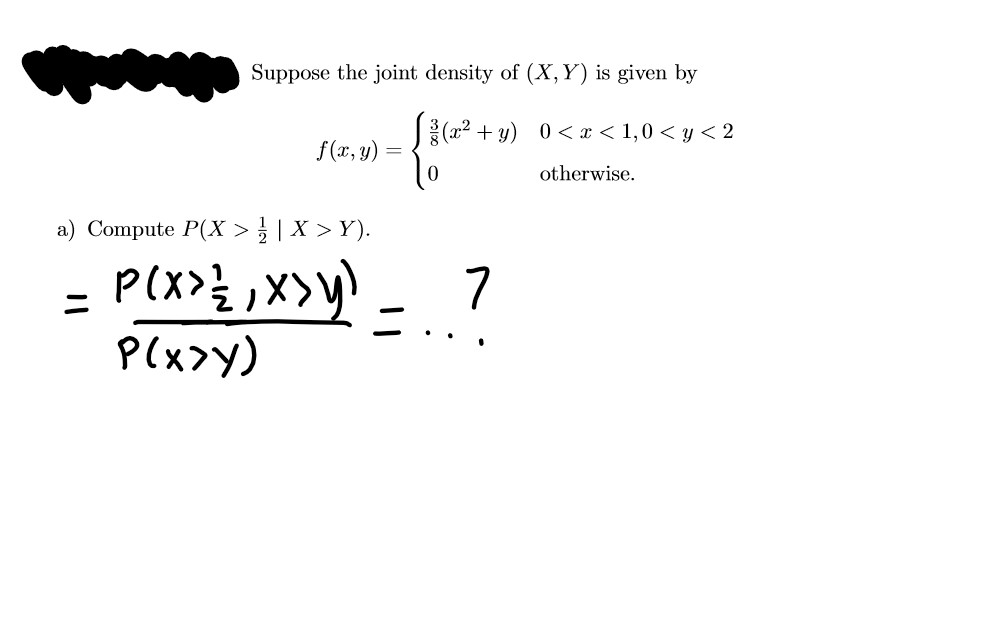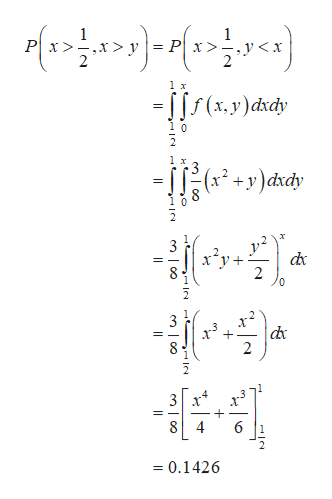# Suppose the joint density of (X,Y) is given by(a2 + y) 0<< x < 1,0 < y < 2f(x, y) =otherwise.a) Compute P(X >} | X > Y).P(x>y)

Question
2 views

For this question i am stuck on P(X>Y) for the conditional probabilityhelp_outlineImage TranscriptioncloseSuppose the joint density of (X,Y) is given by (a2 + y) 0<< x < 1,0 < y < 2 f(x, y) = otherwise. a) Compute P(X >} | X > Y). P(x>y) fullscreen
check_circle

Step 1

From the given information, the joint density function for X and Y is,

Step 2help_outlineImage Transcriptionclose= [[f (x,y)dxdy f(+y)dxdy (r. 3 x²y+ dx dx 8 4 = 0.1426 %3! fullscreen

### Want to see the full answer?

See Solution

#### Want to see this answer and more?

Solutions are written by subject experts who are available 24/7. Questions are typically answered within 1 hour.*

See Solution
*Response times may vary by subject and question.
Tagged in

### Other